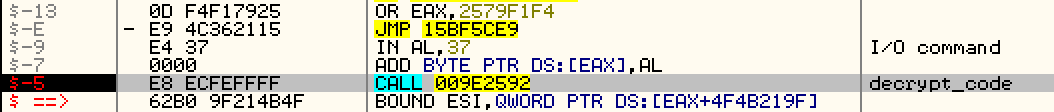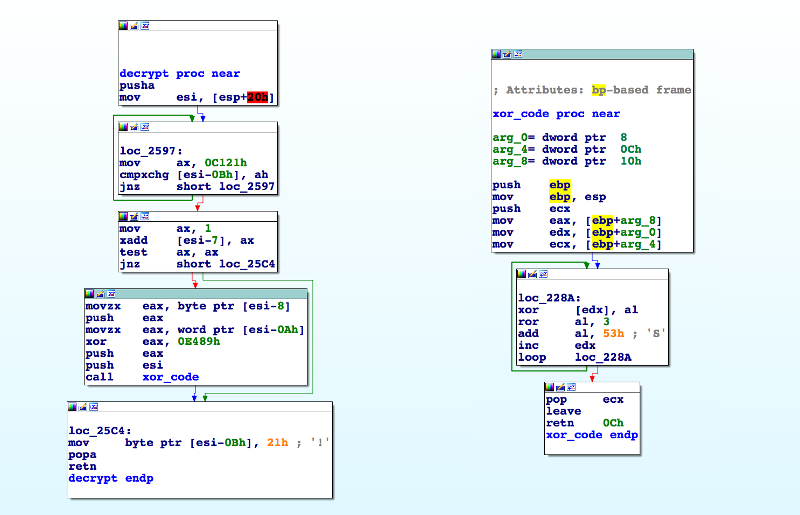After reading the Malwarebytes blog post describing Fobber, a new variant of Tinba, I wanted to have a look at it myself (MD5 `691ce6807925fed03cb61f4add0e5ffd`).

Instead of unpacking all the code at once in memory, Fobber uses a cleverer way to make the analysis much more difficult. Before any code can be executed, Fobber performs the following:

• Decrypt the function to be executed
• Execute it
• Re-encrypt the function

In this post, let’s have a look on how the decryption routine works. On the screenshot below we see OllyDbg stopped before the decryption function at `0x009E2592` is getting called.The decryption function will use specific bytes preceding this call to perform the decryption. Let’s describe them:

Position Description
`- 0xB` Mutex: either `0x21` (free) or `0xC1` (occupied)
`- 0xA..0x9` Code length
`- 0x8` XOR key
`- 0x7` Either 1 (decrypted) or 0 (encrypted)
`- 0x6` Not used
`- 0x5..0x1` Call to decrypt function
`0x0` First byte of encrypted code

If we want to match these field to the above code we obtain:

``````Mutex: 0x21
Key: 0x37
Size 0x9c bytes
Encrypted: 0x0
``````

The size is not directly used but it is first XORed with the constant `0x0E489`, so `0xE415 ^ 0x0E489 = 0x9C`

The mutex is `0x21` meaning that no other thread is decrypting it.

Now let’s have a look at the decryption function in IDA:In `loc_2597` the decrypt function waits until the mutex is free (value `0x21`), then it adds 1 to the -7th byte and checks that the the previous value was `0x0` (encrypted), if so, it continues by pushing the XOR key and the computed size to the stack.

Function `xor_code` performs the actual decryption. This function loops from 0 to `size` and for each byte code performs the following:

• XOR the current code byte with the XOR key
• Compute a new XOR key for the next step by rotating the bits of the XOR key 3 position to the right (ROR) Once finished it foes back

To simplify this process I wrote a small python script performing the steps described above. You can either use it directly on a dump of the injected memory or adapt it to be loaded in IDA debugger. The script uses pwntools library to dump assembly code to the console.

``````import re
import struct
from pwnlib.asm import disasm
from pwnlib.context import context

context(arch='i386', os='windows', endian='big', word_size=32)

# Injected memory dump file
f = open("./data/dump_009E0000.mem", "rb")

def decrypt_section(key, size, data, start, end):
ror = lambda val, r_bits, max_bits: \
((val & (2 ** max_bits - 1)) >> r_bits % max_bits) | \
(val << (max_bits - (r_bits % max_bits)) & (2 ** max_bits - 1))

tmpkey = key
for i in range(start, end):
b = data[i]
b = ((b ^ tmpkey) & 0xFF)
tmpkey = ror(tmpkey, 0x3, 8)
tmpkey += 0x53
tmpkey &= 0xFF
data[i] = b

# Write ASM code to the console
print disasm(data)

total_length = len(d)
print "Total length %s bytes" % hex(total_length)
count = 0

# Match where encrypted functions begins using this regex
for m in re.finditer('\x21[\x00-\xFF]{5}\xe8[\x00-\xFF]{4}', d):
print "Encrypted code section found at %s" % hex(m.start())
size = struct.unpack('<H', d[m.start() + 1:m.start() + 3])
size ^= 0x0e489
if size > total_length:
print "Wrong match, size bigger then binary data"
continue
key = struct.unpack('B', d[m.start() + 3:m.start() + 4])
encrypted = struct.unpack('B', d[m.start() + 4:m.start() + 5])
if encrypted > 0:
print "Wrong match, encrypted flag must be zero"
continue

print "Key: %s" % hex(key)
print "Size: %s bytes" % hex(size)
print "Encrypted: %s" % hex(encrypted)

decrypt_section(key, size, d, m.end(), m.end() + size)
count += 1

print "-" * 30
print "Found %d references!" % count
# Save the decrypted code to a file
wf = file("./data/decrypted.bin", "wb")
wf.write(d)
print "Written %d bytes to %s" % (len(d), wf.name)
``````